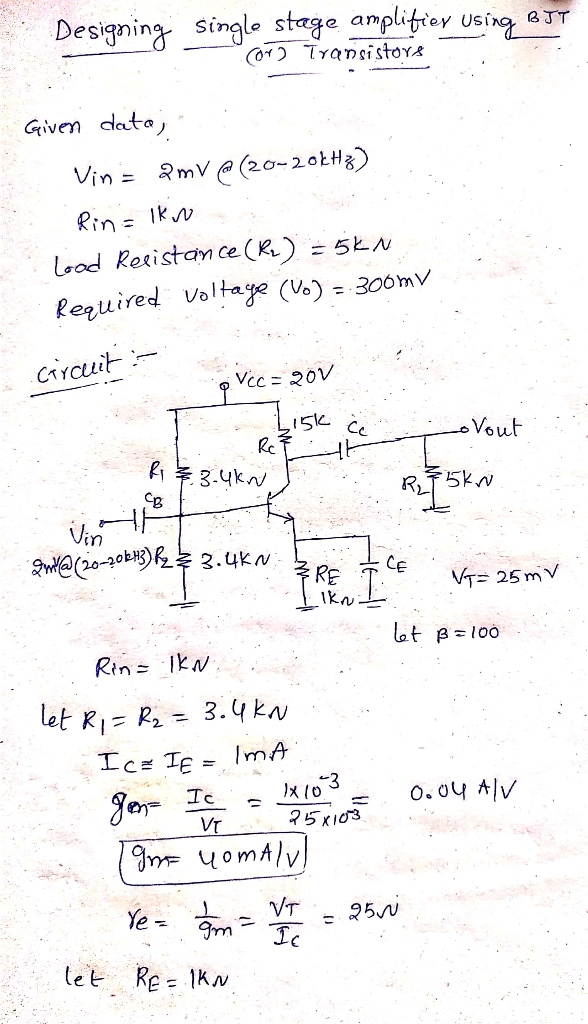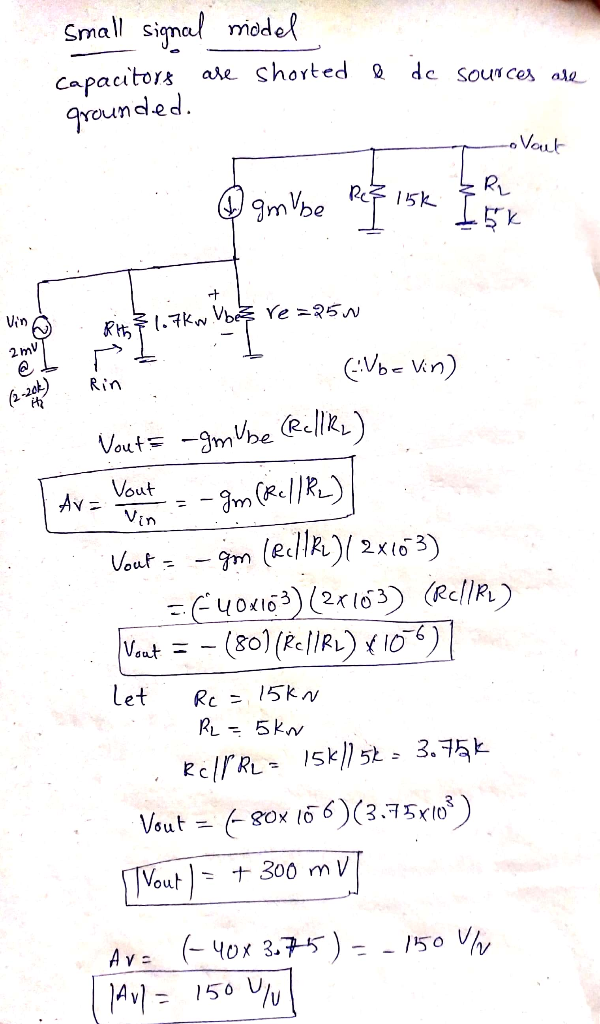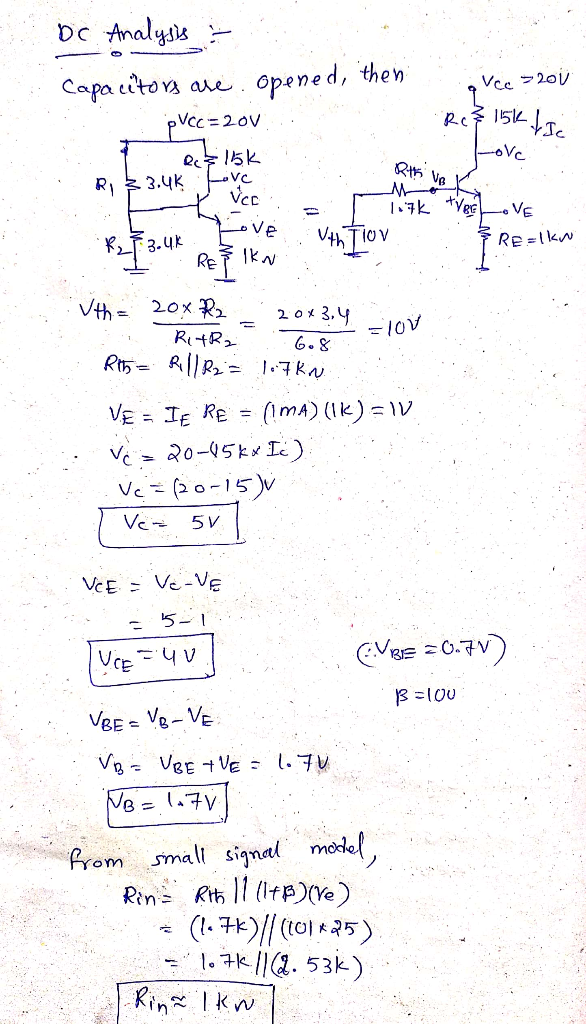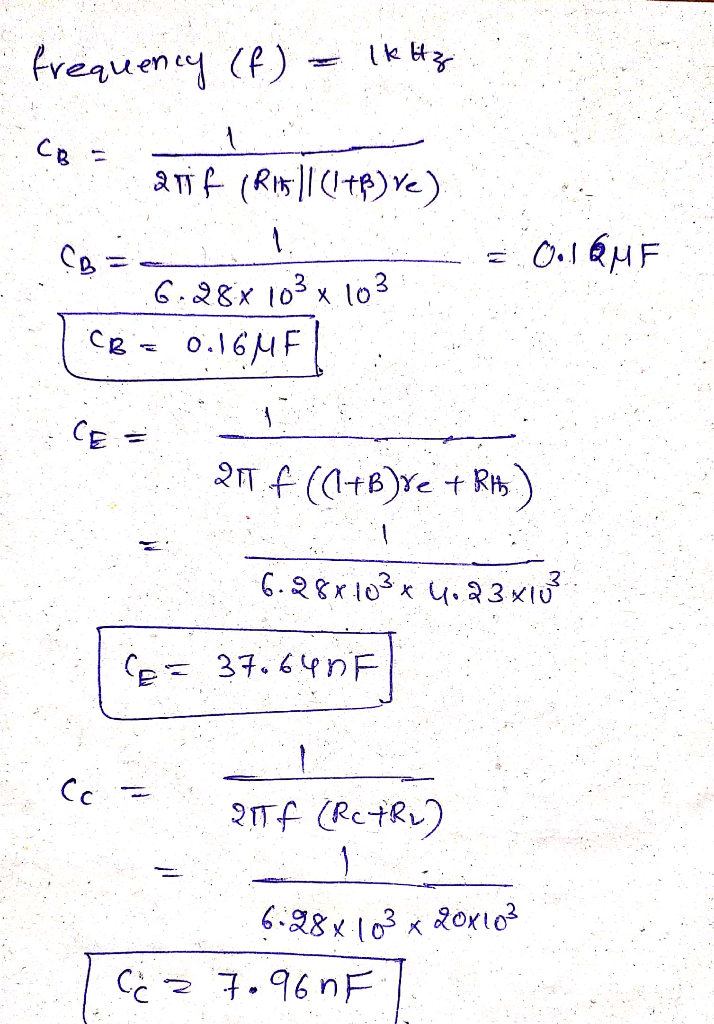##### Question

In: Electrical Engineering

# Design a complete single-stage amplifier using transistor to amplifier a sine-wave voltage signal with an amplitude...

Design a complete single-stage amplifier using transistor to amplifier a sine-wave voltage signal with an amplitude of 2 mV, frequency range from 20-20kHz and internal resistance of 1k ohm. the output of the amplifier supplies to a load of 5k ohm and the required voltage amplitude around 300 mV.

## Solutions

##### Expert Solution

Designing single stage amplifier with BJT transistor:## Related Solutions

##### Design a three-stage operational amplifier at the transistor level using BJT technology. Your design should include...
Design a three-stage operational amplifier at the transistor level using BJT technology. Your design should include a differential input stage with large input resistance, a gain stage, and an output stage with low input resistance. Justify your design choices. Use the op-amp you designed to create an inverting amplifier using negative feedback with a given gain, A = −Rf/Ri . Provide a characterization of your op-amp design which includes input resistance, output resistance, input bias currents, input offset current, input...
##### One transistor amplifier BJT 1.Design a single transistor amplifier BJT with appropriate coupling and bypassing capacitors....
One transistor amplifier BJT 1.Design a single transistor amplifier BJT with appropriate coupling and bypassing capacitors. 2.Draw the DC equivalent circuit and find the Q point. Draw the AC equivalent circuit and find parameters of your full amplifier circuit (gain and input resistance) . 3.Simulate the FULL amplifier circuit in DC state, indicate the Q point on the diagram and compare with theoretical results. 4.Simulate the FULL amplifier circuit using appropriate sin input signal(s). Using the simulation results from the...
##### In the following graph of a sine wave, the Y-axis is voltage (Volts) and the X-axis is time seconds). What is the voltage amplitude?
In the following graph of a sine wave, the Y-axis is voltage (Volts) and the X-axis is time seconds). What is the voltage amplitude? What is the phase shift in seconds?What is the phase shift in degrees?What is the phase shift in radians?
##### Design a sine wave generator with adjustable frequency and amplitude. Show circuit diagram please!
Design a sine wave generator with adjustable frequency and amplitude. Show circuit diagram please!
##### a) As an Engineer you are to design a single stage JFET Amplifier. Explain in your...
a) As an Engineer you are to design a single stage JFET Amplifier. Explain in your own words its operation , identifying each components and their functions . b) With your knowledge and understanding in MOSFET , explain electrically with graph supporting your answers , the three regions of operation of a MOSFET. please answer all.thank you.
##### Design a single stage BJT amplifier with the following constraints: This involves the following: -DC and...
Design a single stage BJT amplifier with the following constraints: This involves the following: -DC and AC analysis and the frequency response of the amplifier. -Use a 2N2222 transistor -Power supply (VCC): 6 V -An input impedance near to 3 kΩ -Overall midband voltage gain of -30 V/V -A load resistance of 2 kΩ -The input signal has an RSignal of 500 Ω -Choose a good Qpoint and select the coupling and bypass capacitors to obtain an fL-3dB of 100...
##### 1. Imagine a sine wave on the oscilloscope screen that had an amplitude of 4V and...
1. Imagine a sine wave on the oscilloscope screen that had an amplitude of 4V and a period of 2ms. If you turn the time/division knob to something larger, will one number change, both, or neither? If one does change, does it increase or decrease? 2. When measuring amplitude of a wave, why is it useful to measure from the bottom to the top of the wave? 3. What is the mechanical audio signal that the microphone receives changed to...
##### 1. A sinusoidal voltage wave on a transmission line has real amplitude, V_0. The wave is...
1. A sinusoidal voltage wave on a transmission line has real amplitude, V_0. The wave is expressed in phasor form as V_s(z) = V_0*e^(-alpha*z)*e^(-j*(beta)*z) a. In which direction does this wave propagate and why? b. Express the given wave in real instantaneous form. c. The parameters of the line, operating at ω= 6.0×10^8 rad/s are L = 0.350 μH/m, C = 40.0 pF/m, G = 0 and R = 15.0 Ω/m. Find α,β,λ, andZ_0 (in magnitude and phase). d. Calculate...
##### Design a differential amplifier using three 2N3904 transistors. Use the third transistor as acurrent source device....
Design a differential amplifier using three 2N3904 transistors. Use the third transistor as acurrent source device. The differential transistors are to be biased at 10v and 10mA. Use Vcc of your choice and differential gain at 1KHz is to be 50.
##### with a voltage range of 0.874 and -0.855 how can i design a inverting amplifier using...
with a voltage range of 0.874 and -0.855 how can i design a inverting amplifier using standard resistors to produce a voltage output range of -10 to 10 volts?